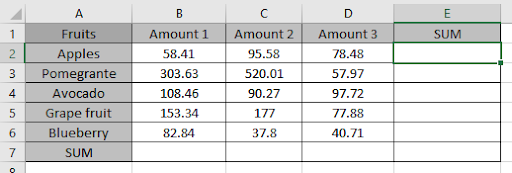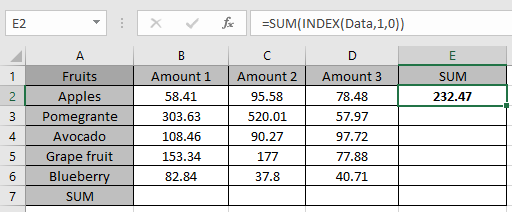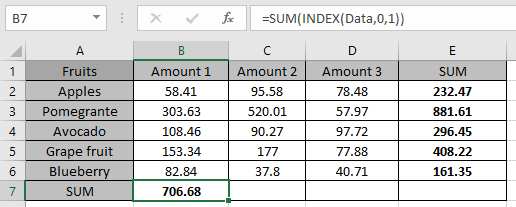# SUM range with INDEX in Excel

In this article, we will learn about how to get the SUM of range with INDEX function in Excel.

The INDEX function returns the values of the referred cell of the data.
Syntax:

=INDEX (data, row_num, column_num)

data : array
row_num : row number of the data. Returns whole column, if row_num = 0
column_num : column number of the data. Returns whole row, if row_num = 0

Let’s understand this function using it an example.Here we have a list of Fruits and their different prices.
We need to find the SUM of column wise and row wise.
First Row wise.

Now we need to have the sum of all three amounts of Apples.

Use the formula:

=SUM(INDEX(Data,1,0))

Data : B2:D6 via define name
1 : First row of data array
0 : returns the values of whole row

Explanation:
INDEX function returns all the values of the first row of the data. The formula drops down to do this
=SUM( {58.41, 95.58, 78.48} )Here you got the Sum of first row

Now replace the row number with 2, 3, 4 & 5 one by one to get the sum of all rows in different cells.
As you can see we got the sum of the rows.

Now we will do it Column wise.

Use the formula:

=SUM(INDEX(Data,0,1))

Data : B2:D6 via define name
0 : returns the values of whole column
1 : First column of data array

Explanation:
INDEX function returns all the values of the first column of the data. The formula drops down to do this
=SUM( {58.41; 303.63; 108.46; 153.34; 82.84})
Here you got the Sum of first row

Now replace the row number with 2 & 3 one by one to get the sum of all columns of Data array in different cells.As you can see we got the sum of the columns of the Data array using Excel SUM and INDEX function

Hope you understood how to get the SUM of range using INDEX function in Excel. Explore more articles on Excel cell reference function here. Please feel free to state your query or feedback for the above article.

Terms and Conditions of use

The applications/code on this site are distributed as is and without warranties or liability. In no event shall the owner of the copyrights, or the authors of the applications/code be liable for any loss of profit, any problems or any damage resulting from the use or evaluation of the applications/code.